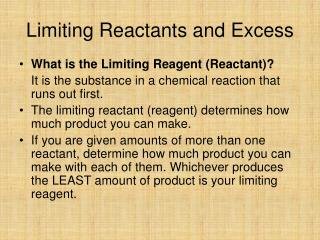DownloadDownload PresentationLimiting Reactants and Excess

# Limiting Reactants and Excess

Download Presentation## Limiting Reactants and Excess

- - - - - - - - - - - - - - - - - - - - - - - - - - - E N D - - - - - - - - - - - - - - - - - - - - - - - - - - -
##### Presentation Transcript

1. Limiting Reactants and Excess • What is the Limiting Reagent (Reactant)? It is the substance in a chemical reaction that runs out first. • The limiting reactant (reagent) determines how much product you can make. • If you are given amounts of more than one reactant, determine how much product you can make with each of them. Whichever produces the LEAST amount of product is your limiting reagent.

2. Practice Problems Consider the reaction: 2 Al + 3 I2 2 AlI3 Determine the limiting reagent of the product, aluminum iodide, if one starts with: a). 1.20 moles of Al and 2.40 moles of iodine • Al • I2

3. 1.20 moles of Al 2 moles AlI3 1.20 moles AlI3 • 2 moles Al • 2.40 moles I2 2 moles AlI3 1.60 moles AlI3 3 moles I2 • Al is limiting reactant

4. What is the limiting Reactant with 1.20 grams of Al and 2.40 grams of iodine? A 1.20g Al B 2.40 g I2 1.20 g Al 1mol Al 2 mol AlI3 .0445 mols AlI3 26.9815 g 2 mol Al 2.40 g I2 1 mol I22 mol AlI3 .00630 mols AlI3 253.8089 g 3 mole I2 I2 is limiting Reactant! B

5. How many grams of excess reactant will remain? 2.40g I2 1 mol I22 mols Al 26.9815g 253.8089g 3 mole I2 1 mol Al .0.170 g of Al reacted Started with 1.20g -0.170g reacted 1.03 g in excess

6. 15.00 g aluminum sulfide and 12.00 g of water react until the limiting reagent is used up. Here is the balanced equation for the reaction. Al2S3 + 6 H2O  2 Al(OH)3 + 3 H2S a.) What is the maximum mass of H2S which can be formed from these reagents ? • 10.21g • 11.35 g 15.00g Al2S3 1 mol Al2S3 3 mols H2S 34.08 g 150.16167g 1 mol Al2S3 1 mol H2S 10.21g H2S 12.00g H2O 1 mol H2O 3 mols H2S 34.08 g 18.008g 6 mol H2O1 mol H2S 11.35g H2S A. 10.21g

7. What is the limiting Reactant? • A . H2O • B. Al2S3 • B! • How much of the excess reactant is used up? • A, 1.200g • B. 12.00 g • C. 10.84 g 15.00g Al2S3 1 mol Al2S36 mols H2O 18.08 g 150.16167g 1 mol Al2S3 1 mol H20 10.84 g used up

8. Percent Yield Percent Yield = (Actual Yield / Theoretical Yield) * 100 • Theoretical Yield is the amount that your stoichiometric calculations have predicted. • Actual Yield is what you actually produced in an experiment.

9. Example: In the reaction between nitrogen gas and hydrogen gas producing ammonia: a.) Ifyou start with 14 grams of N2 and 6.0 grams of H2, what will be the limiting reagent and the theoretical yield? b.) If 10. grams of the product was formed, what would be the percent yield?

10. Stoichiometry with Solutions • Just like other problems but use Moles = Molarity X Liters to get moles, or Liters = Moles/Molarity to find the volume of a solution needed in a reaction.

11. How many grams of aluminum will be required to completely replace copper from a 208 mL of a 0.100M solution of copper(II) chloride? 2 Al + 3 CuCl2 2 AlCl3 + 3 Cu Answer = 0.374 g Al

12. How many milliliters of a 3.40M CuSO4 solution will be needed to react with 510.0 g of AgCl in the following reaction? AgCl + CuSO4 Ag2SO4 + CuCl2 Answer = 523 mL